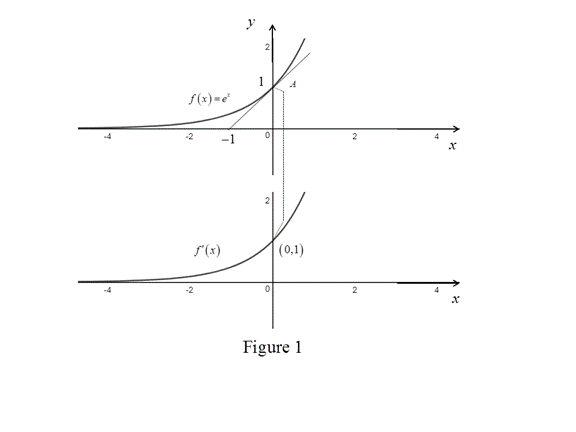# The graph of f ( x ) and then f ′ ( x ) below it.### Single Variable Calculus: Concepts...

4th Edition
James Stewart
Publisher: Cengage Learning
ISBN: 9781337687805### Single Variable Calculus: Concepts...

4th Edition
James Stewart
Publisher: Cengage Learning
ISBN: 9781337687805

#### Solutions

Chapter 2.7, Problem 15E
To determine

## To sketch: The graph of f(x) and then f′(x) below it.

Expert Solution

The formula for f(x) from its graph is, f(x)=ex.

### Explanation of Solution

The given function is f(x)=ex.

From the graph of f(x), it is clear that the slope is always positive. Thus, the derivative of the graph is always positive.

Draw the tangent to the graph at x=0 and the slope of the graph is computed as follows,

m=0110=11=1

Recall the fact that, the slope of the function at the point x=a is equal to the derivative of the function at that point.

Therefore, f(x)=1 at x=0.

Thus, the graph of the function f(x) passes through the point (0,1).

From the point A to left, the slope is increasing slowly. Thus the graph of the function f(x) in this section will rise slowly.

From the point A to right, the slope increases rapidly. Thus the graph of the function f(x) in this section will rise highly.

Graph:

Use the online graphing calculator to draw the graph of f(x)=ex and use the above information to trace the graph of f(x) as shown below in Figure 1.Observation:

From the Figure 1, it is observed that the graph of the derivative function seems to be a natural exponential function.

Thus, the derivative formula is, f(x)=ex.

### Have a homework question?

Subscribe to bartleby learn! Ask subject matter experts 30 homework questions each month. Plus, you’ll have access to millions of step-by-step textbook answers!